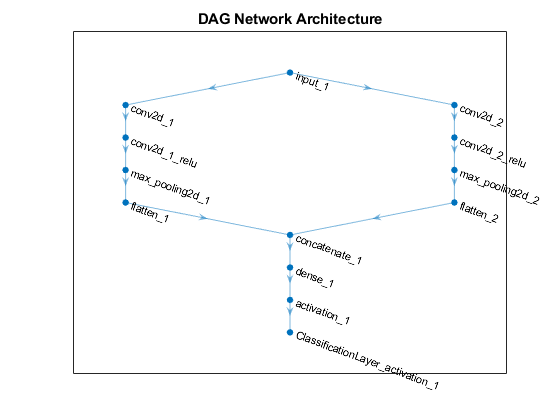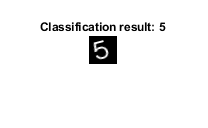Documentation

# importKerasNetwork

Import a pretrained Keras network and weights

## Syntax

``net = importKerasNetwork(modelfile)``
``net = importKerasNetwork(modelfile,Name,Value)``

## Description

example

````net = importKerasNetwork(modelfile)` imports a pretrained TensorFlow™-Keras network and its weights from `modelfile`.This function requires Deep Learning Toolbox™ Importer for TensorFlow-Keras Models support package. If this support package is not installed, the function provides a download link.```
````net = importKerasNetwork(modelfile,Name,Value)` imports a pretrained TensorFlow-Keras network and its weights with additional options specified by one or more name-value pair arguments.For example, `importKerasNetwork(modelfile,'WeightFile',weights)` imports the network from the model file `modelfile` and weights from the weight file `weights`. In this case, `modelfile` can be in HDF5 or JSON format, and the weight file must be in HDF5 format.```

## Examples

collapse all

Download and install the Deep Learning Toolbox Importer for TensorFlow-Keras Models support package.

Type `importKerasNetwork` at the command line.

`importKerasNetwork`

If the Deep Learning Toolbox Importer for TensorFlow-Keras Models support package is not installed, then the function provides a link to the required support package in the Add-On Explorer. To install the support package, click the link, and then click . Check that the installation is successful by importing the network from the model file `'digitsDAGnet.h5'` at the command line. If the required support package is installed, then the function returns a `DAGNetwork` object.

```modelfile = 'digitsDAGnet.h5'; net = importKerasNetwork(modelfile)```
```Warning: Saved Keras networks do not include classes. Classes will be set to categorical(1:N), where N is the number of classes in the classification output layer of the network. To specify classes, use the 'Classes' argument. net = DAGNetwork with properties: Layers: [13×1 nnet.cnn.layer.Layer] Connections: [13×2 table]```

Specify the file to import. The file `digitsDAGnet.h5` contains a directed acyclic graph convolutional neural network that classifies images of digits.

`modelfile = 'digitsDAGnet.h5';`

Import the network.

`net = importKerasNetwork(modelfile)`
```Warning: Saved Keras networks do not include classes. Classes will be set to categorical(1:N), where N is the number of classes in the classification output layer of the network. To specify classes, use the 'Classes' argument. ```
```net = DAGNetwork with properties: Layers: [13×1 nnet.cnn.layer.Layer] Connections: [13×2 table] ```

Plot the network architecture.

```figure plot(net); title('DAG Network Architecture')```Specify the network and the weight files to import.

```modelfile = 'digitsDAGnet.json'; weights = 'digitsDAGnet.weights.h5';```

This is a directed acyclic graph convolutional neural network trained on the digits data.

Import network architecture and import the weights from separate files. The .json file does not have an output layer or information on the cost function. Specify the output layer type when you import the files.

```net = importKerasNetwork(modelfile,'WeightFile',weights, ... 'OutputLayerType','classification')```
```Warning: Saved Keras networks do not include classes. Classes will be set to categorical(1:N), where N is the number of classes in the classification output layer of the network. To specify classes, use the 'Classes' argument. ```
```net = DAGNetwork with properties: Layers: [13×1 nnet.cnn.layer.Layer] Connections: [13×2 table] ```

Specify the model file.

`modelfile = 'digitsDAGnet.h5';`

Specify class names.

`classNames = {'0','1','2','3','4','5','6','7','8','9'};`

Import the Keras network with the class names.

`net = importKerasNetwork(modelfile,'Classes',classNames);`

```digitDatasetPath = fullfile(toolboxdir('nnet'),'nndemos','nndatasets', ... 'DigitDataset'); I = imread(fullfile(digitDatasetPath,'5','image4009.png'));```

Classify the image using the pretrained network.

`label = classify(net,I);`

Display the image and the classification result.

```figure imshow(I) title(['Classification result: ' char(label)])```## Input Arguments

collapse all

Name of the model file containing the network architecture, and possibly the weights, specified as a character vector or a string scalar. The file must be in the current folder, in a folder on the MATLAB® path, or you must include a full or relative path to the file.

If `modelfile` includes

• The network architecture and weights, then it must be in HDF5 (`.h5`) format.

• Only the network architecture, then it can be in HDF5 or JSON (`.json`) format.

If `modelfile` includes only the network architecture, then you must supply the weights in an HDF5 file, using the `'WeightFile'` name-value pair argument.

Example: `'digitsnet.h5'`

Data Types: `char` | `string`

### Name-Value Pair Arguments

Specify optional comma-separated pairs of `Name,Value` arguments. `Name` is the argument name and `Value` is the corresponding value. `Name` must appear inside quotes. You can specify several name and value pair arguments in any order as `Name1,Value1,...,NameN,ValueN`.

Example: `importKerasNetwork(modelfile,'OutputLayerType','classification','Classes',classes)` imports a network from the model file `modelfile`, adds an output layer for a classification problem at the end of the Keras layers, and specifies `classes` as the classes of the output layer.

Name of file containing weights, specified as a character vector or a string scalar. `WeightFile` must be in the current folder, in a folder on the MATLAB path, or you must include a full or relative path to the file.

Example: `'WeightFile','weights.h5'`

Type of the output layer that the function appends to the end of the imported network architecture when `modelfile` does not specify a loss function, specified as `'classification'`, `'regression'`, or `'pixelclassification'`. Appending a `pixelClassificationLayer` object requires Computer Vision Toolbox™.

Example: `'OutputLayerType','regression'`

Size of the input images for the network, specified as a vector of two or three numerical values corresponding to `[height,width]` for grayscale images and `[height,width,channels]` for color images, respectively. The network uses this information when the `modelfile` does not specify the input size.

Example: `'ImageInputSize',[28 28]`

Classes of the output layer, specified as a categorical vector, string array, cell array of character vectors, or `'auto'`. If you specify a string array or cell array of character vectors `str`, then the software sets the classes of the output layer to `categorical(str,str)`. If `Classes` is `'auto'`, then the function sets the classes to `categorical(1:N)`, where `N` is the number of classes.

Data Types: `char` | `categorical` | `string` | `cell`

## Output Arguments

collapse all

Pretrained Keras network, returned as one of the following:

## Compatibility Considerations

expand all

Not recommended starting in R2018b

 Keras: The Python Deep Learning library. https://keras.io.